Select Page

# TimeSinceSignal formulas

Optuma Forums Optuma Scripting TimeSinceSignal formulas

Viewing 5 posts - 1 through 5 (of 5 total)
• Author
Posts
• #48071

The TimeSinceSignal function is very useful to determine how long has elapsed since a technical event (see here and here for some examples).

Here’s a variation which counts the number of trading days since two moving averages crossed, calculating a positive number when crossing above and a negative number when crossing below. The example is for the 20EMA crossing the 50SMA:

The script can be added to a watchlist column and also a Show View to display the results (note the Show View properties have been set to a Dot plotstyle, with a positive/negative colour scheme):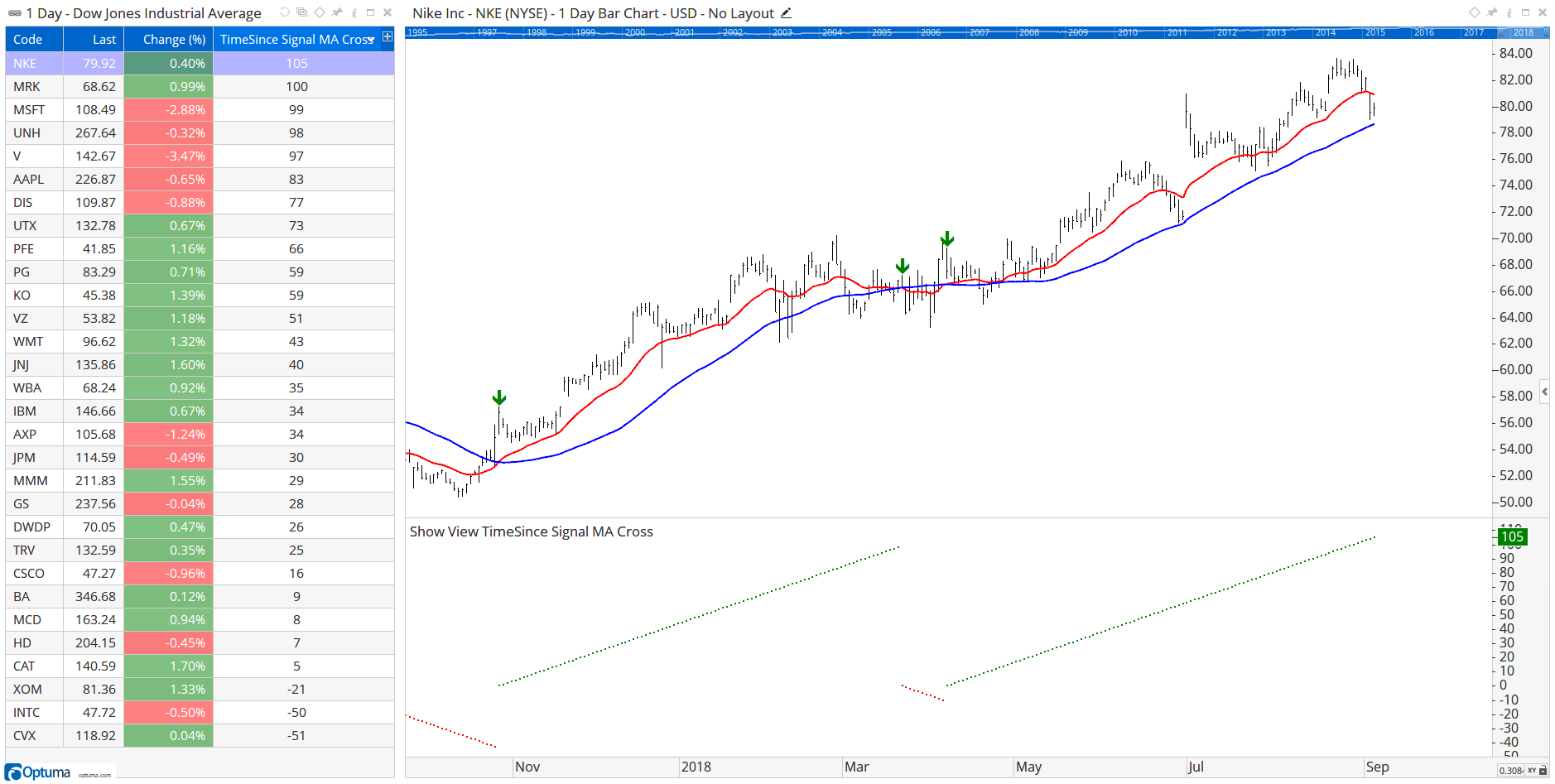So NKE crossed above 105 days ago, and CVX crossed below 51 days ago.

Also note that you can count in calendar days or weeks by changing the Units property in the TimeSinceSignal function: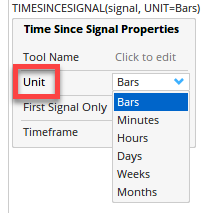###### 3 users thanked author for this post.Andrew,Arthur Hill, CMT,Mathew
#48087

To calculate the time since the signal before last use the following (note: this will return calendar days), in this case RSI crossing above 70: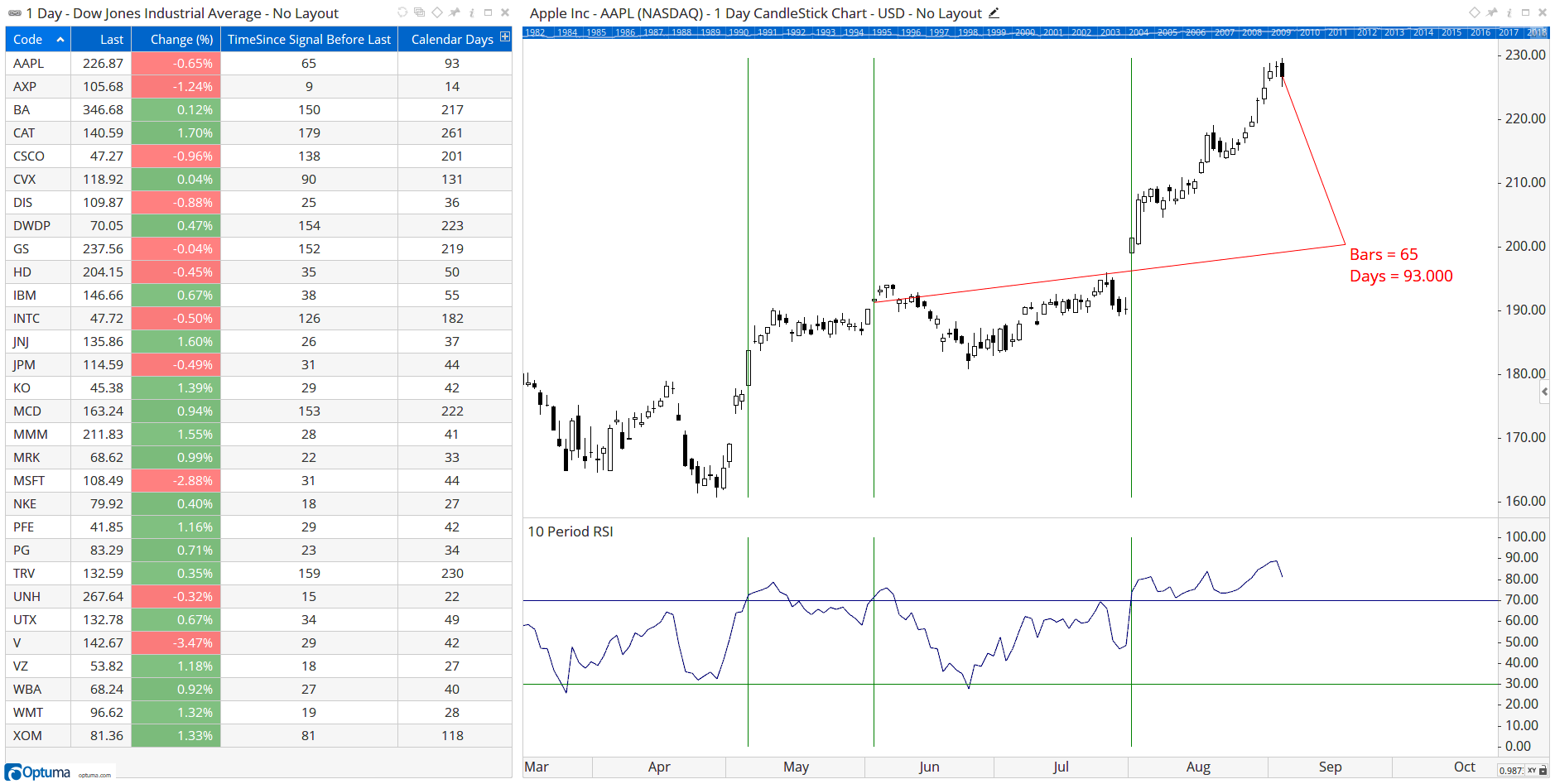#51863

To calculate the time since the current closing price was that high (in trading days):

To calculate the time since the current closing price was that low (in calendar days):

To show the date use the following BARDATE() function, and set the watchlist Column Type to Date: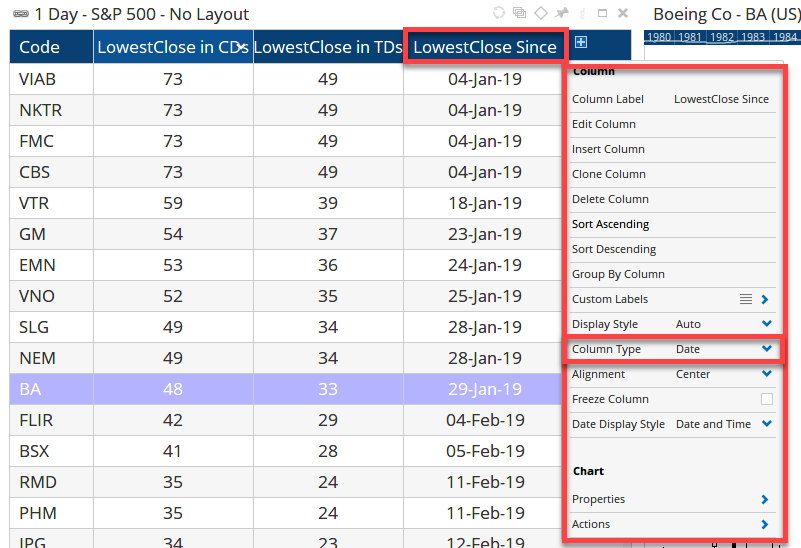#58335

Thanks everyone at Optuma for all of the help with the scripting. These forums really are incredibly useful.

I have a basic script where i am looking for ADX > 14 and DM+ > DM-. I am trying to create a TimeSinceSignal script to return the number of days since these conditions were met. Strangely the script is returning 0 for all stocks where the signal is currently live, and a value for stocks where it is not.

I see the logic in that the script is returning the number of days since the signal ended, how would I correct this to return the number of days since it was triggered?

Many thanks,

Dean

#58337

Hi Dean,

I think the issue is that you may be counting since the condition was last true – not how long it has been true, hence the zeroes for those where currently true. It’s a bit confusing, but instead you need to count how long it’s been since the condition was last false. Remember that a value of 1 is true and 0 is false, so this will show how long it has been true by counting the days since it was false:

This list of FTSE100 stocks shows when the V1 condition is true in blue on the chart, with the green showing the time counts. So OCDO has been positive for 51 days, whereas the ADX False column shows how long it has been false (in other words how long since it’s been true) i.e. 28 days for NG. Hope that makes sense!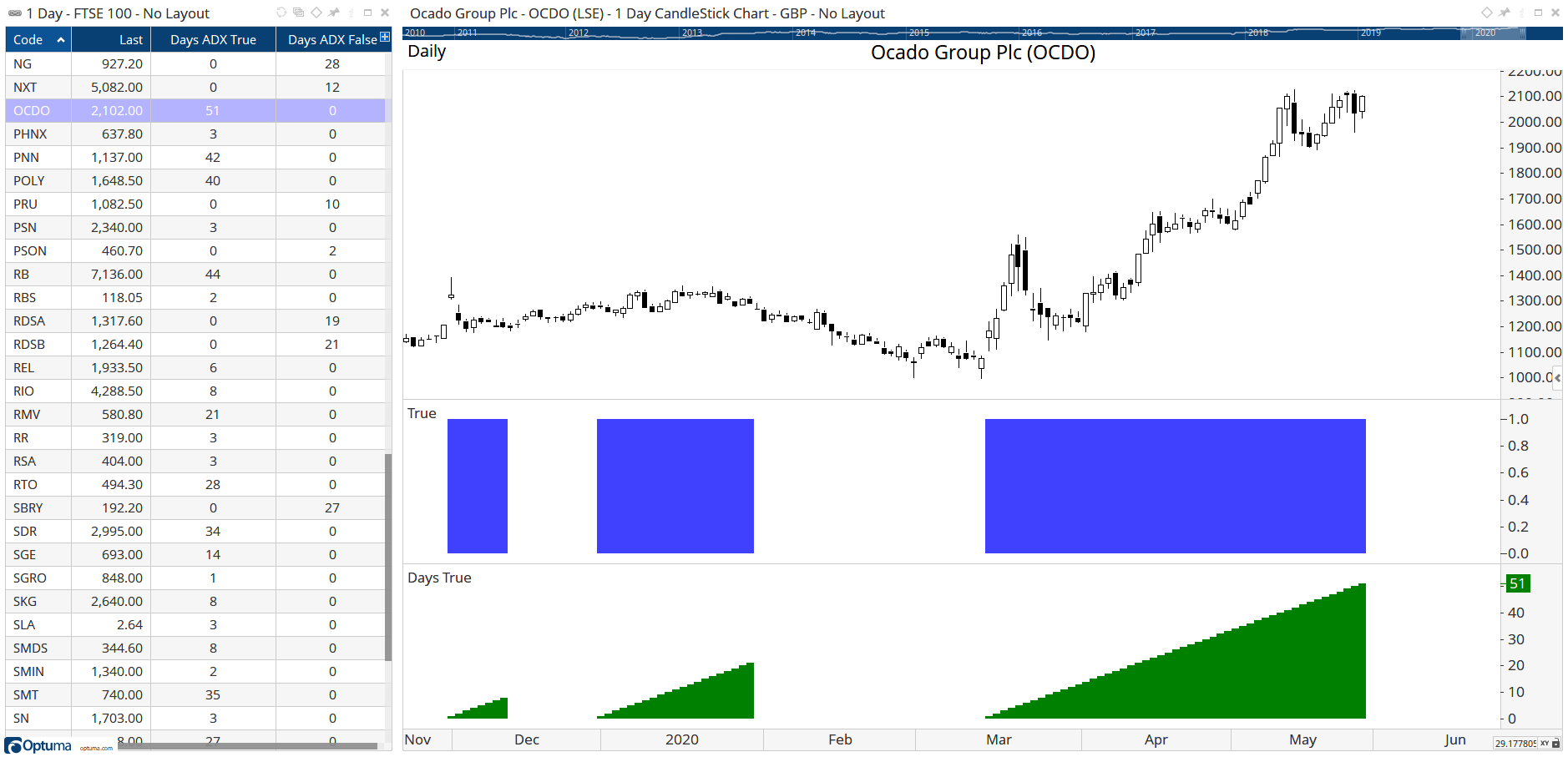###### 1 user thanked author for this post.Thomas
Viewing 5 posts - 1 through 5 (of 5 total)
• You must be logged in to reply to this topic.